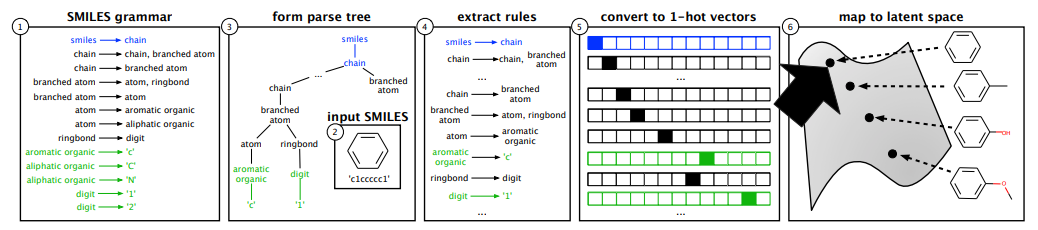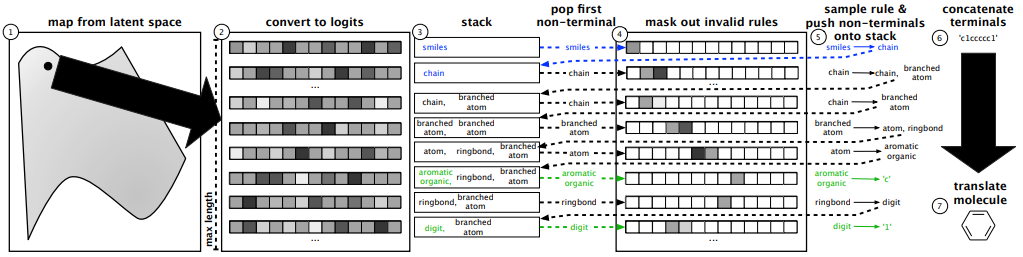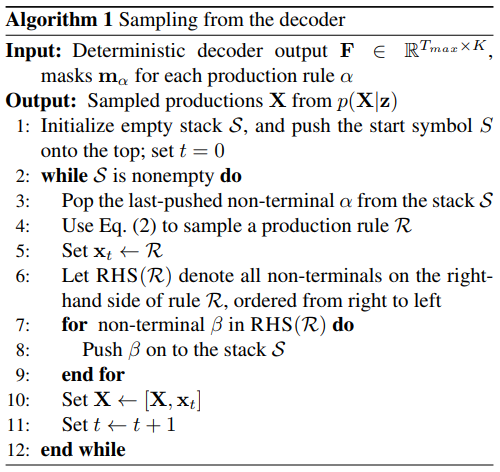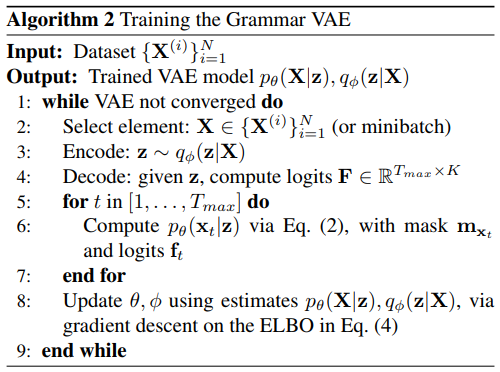# Grammar Variational Autoencoder

## WHY?

Generative models of discrete data with particular structure (grammar) often result invalid outputs. Grammar Variational Autoencoder(GVAE) forces the decoder of VAE to result only valid outputs.

## WHAT?

Particular structure of data can be formulated with context-free grammars(CFG). Data with defined CFG can be represented as a parse tree.

G = (V, \Sigma, R, S)Encoder uses the knowledge of rules explicitly. 1) Explicit rules of data are defined. 2) Data can be represented as parse tree and rules in data can be extracted. 3) Rules can be converted into 1-hot vectors. 4) Data represented with 1-hot vectors of rules can be map to latent space with convolution neural network.Decoder is a recurrent neural network to output sequences of discrete tokens. 1) Decoder sequentially output logits of grammar. 2) A stack(last-in first-out) is used to keep track of parsing of data (starts with start token). 3) Following the order of stack, put masks on the logit with unmasked part strictly follow the grammar. Masking process ensures decoder to only result gramatically valid output. 4) Reconstruct data from parse tree. Detailed algorithm is as follows.Training phase is the same as that of variational autoencoder with described encoder and decoder.

## So?

The experiment of GVAE was performed on data of arithmetic expressions and that of molecules. Not only GVAE results valid outputs, it captured more meaningful latent space than character variational autoencoder(CVAE).

## Critic

Seems naive but inspirational. Some data have strict rules to follow, and there’s no reason not to use them. Rule2vec?

Powered by aiden